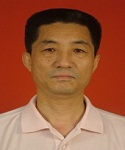2003 博士，四川大学

1987 硕士，华东师范大学

2003年至今       教授，山东大学

1996年至2003 教授，滨州学院

1992年至1996 副教授，滨州学院

1987年至1992 讲师，滨州学院

1. Jianguo Si, Quasi-periodic solutions of a non-autonomous wave equations with quasi-periodic forcing, J. Differential Equations 252 (2012) 5274-5360
2. Yi Wang and Jianguo Si, A result on quasi-periodic solutions of a nonlinear beam equation with a quasi-periodic forcing term, Z. Angew. Math. Phys. 63 (2012), 189-190
3. M.Zhang, Jianguo Si, C^{\infty}-solutions for a class of generalized Feigenbaum’s functional equations, Science in China, Series A-Mathematics, 52 (2009), 1589-1828
4. Hanssmann H, Jianguo Si, Quasi-periodic solutions and stability of the equilibrium for quasi-periodically forced planar reversible and Hamiltonian systems under the Bruno condition, Nonlinearity, 23 (2010), 555-577
5. Jianguo Si, X.L.Li, Small divisors problem in dynamical systems and analytic solutions of the Shabat equation, J. Math. Anal. Appl., 367 (2010), 287-295
6. Jianguo Si, H.Y.Zhao, Small Divisor Problem in Dynamical Systems and Analytic Solutions of a q-Difference Equation with a Singularity at the Origin, Results In Math., 58 (2010), 337-353
7. L.H.Zhang, Jianguo Si, New soliton and periodic solutions of (1+2)-dimensional nonlinear Schrodinger equation with dual-power law nonlinearity, Communication in nonlinear science and numerical simulation, 15 (2010), 2747-2754
8. M. Zhang, Jianguo Si, Quasi-periodic solutions of nonlinear wave equations with quasi-periodic forcing, Physica D ,238 (2009), 2185-2215
9. Jianguo Si,M.Zhang, Construction of convex solutions for the second type of Feigenbaum’s functional equations, Science in China, Series A-Mathematics, 52(2009), 1617-1638 (中文版:《中国科学》, A, 39 (2009), 49-70.)
10. J.Liu, Jianguo Si, Analytic solutions of an iterative differential equation under Brjuno condition, Acta Math. Sinica-English Series, 25 (2009), 1469-1482
11. H.Y.Zhao, Jianguo Si, Analytic solutions of a second-order iterative functional differential equation near resonance, Annales Polonici Math., 96 (2009), 209-226
12. Liu lingxia, JIanguo Si, Analytic solutions of a polynomial-like iterative functional equation near resonance, J. Math. Res. Exp., 29 (2009), 737-744
13. Jianguo Si, Invariant tori and unboundedness for second order differential equations with asymmetric nonlinearities depending on the derivatives, Nonlinear Anal., 67(2007), 3098-3115
14. Jianguo Si, H.Y.Zhao, Local invertible analytic solutions for an iterative differential equation related to a discrete derivatives sequence, J.Math. Anal. Appl., 335 (2007), 428-442
15. Jianguo Si, M.H.Ma, Local invertible analytic solution of a functional differential equation with deviating arguments depending on the state derivative, J.Math. Anal. Appl., 327 (2007), 723-734